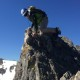# Trip Speed

When going to the mountains, it is always a little bit tricky to calculate how long a trip is going to take. One method of estimating how long a trip will take is the Munter Method of time calculation:

$$t = \frac{d + \frac{e}{100}}{r}$$

Where $t$ is the trip time, $d$ is the distance traveled in kilometers, $e$ is the elevation gain in meters, and $r$ is your rate of travel. Rate of travel depends on your form of travel as well as fitness and group dynamics, however good estimates are:

• Skinning/Walking uphill - 4
• Walking flat/downhill - 6
• Skiing downhill - 10
• Bushwhacking - 2

You can then break your trip into “legs” where each leg has a different activity, and sum the legs together to find your total trip time. For example, if I were planning to ski tour to Uncle Buds Cabin, I know the trail is 5.9 miles one way, with 1,620 feet of elevation gain, or 9.5 kilometers, 500 meters of gain. Calculating each leg:

To the cabin: $t = (9.5 + \frac{500}{100})/4 =$ 3h 38m

Back from the cabin: $t = (9.5 + \frac{500}{100})/10 =$ 1h 27m

For a total round trip time of 5h 5m.

The Munter method can be used for any backcountry activity, but I have most often seen it used for ski touring.

Check out the app at https://chrismarchbanks.com/trip-speed/, and the code at GitHub###### Senior Software Engineer

Skiing, hiking, Prometheus, and all things observability